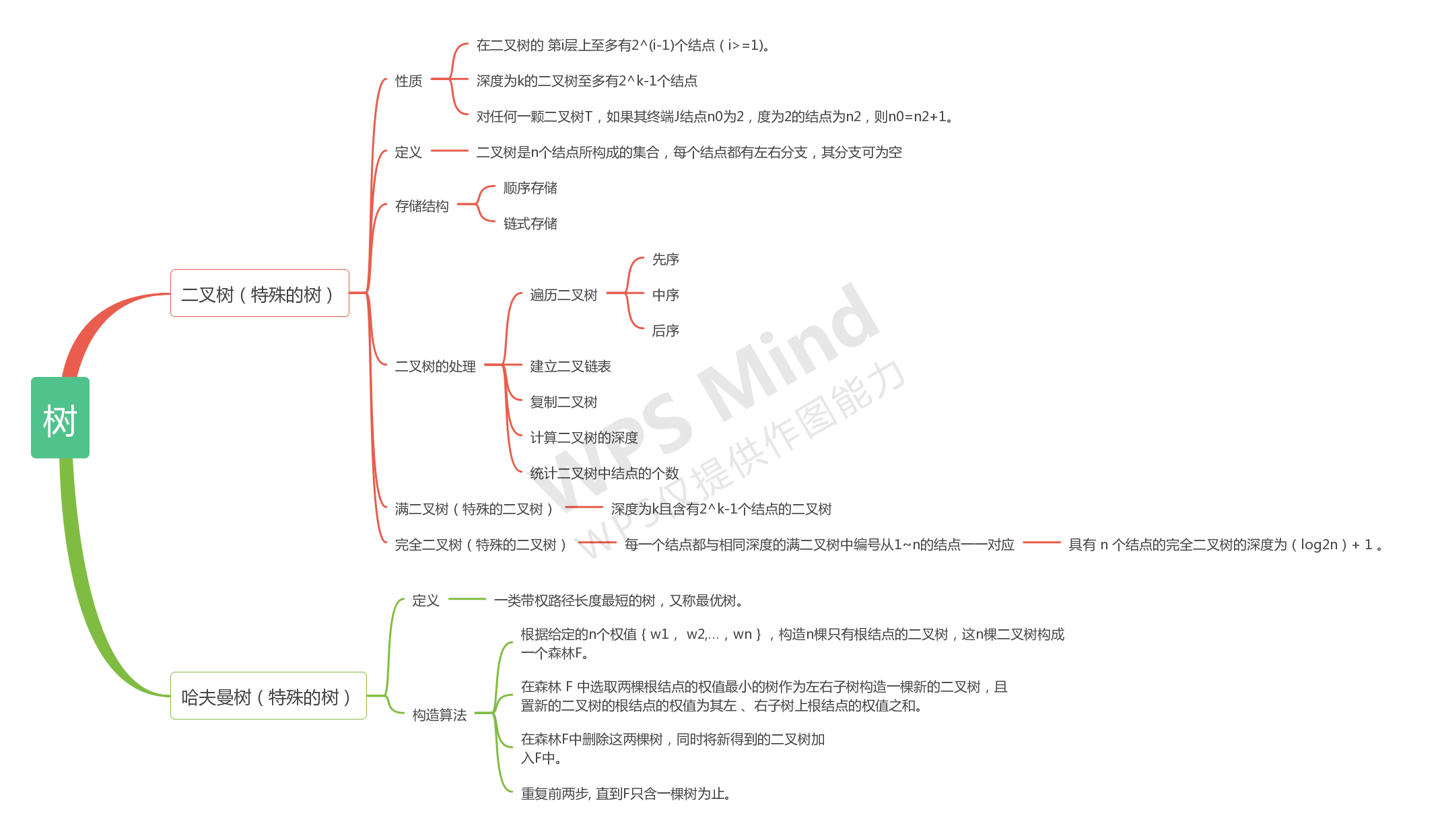# 数据结构：第五章学习小结1.

bool check[n] = {false};//定义bool类型的数组来查找未出现过的结点

2.

2.void LevelOrder(Tree T)
{
queue<int> Q;
int k;
Q.push(T.root);
bool flag = false;
while(!Q.empty())
{
k = Q.front();
Q.pop();
if(T.data[k].lch==-1&&T.data[k].rch==-1)
{
if(!flag)
{
cout << k;
flag = true;
}
else cout << " " << k;
}
else
{
if(T.data[k].lch!=-1) Q.push(T.data[k].lch);
if(T.data[k].rch!=-1) Q.push(T.data[k].rch);
}
}
}

#include<stdio.h>
#define MaxTree 10
#define Null -1     //将Null定义为-1而不能是0，因为数组下标为0的地方仍保存有节点
typedef char ElementType;
typedef int Tree;
struct TreeNode{    //定义二叉树节点
ElementType Data;
Tree Left;
Tree Right;
}T1[MaxTree],T2[MaxTree];
int N,check[MaxTree];   //check数组用于寻找树的根节点

Tree BuildTree(struct TreeNode T[]){
int Root=Null,i;    //刚开始将节点置为空，若为空树的时候可返回Null
char cl,cr;
scanf("%d",&N);
if(N){              //如果不为空树的话
for(i=0;i<N;i++) check[i]=0;    //将check数组置为0
for(i=0;i<N;i++){
scanf("\n%c %c %c",&T[i].Data,&cl,&cr); //把换行符放在前面吃掉前面scanf后的回车，而最后一个scanf不能有回车，一举两得
if(cl!='-'){
T[i].Left=cl-'0';
check[T[i].Left]=1;
}
else
T[i].Left=Null;

if(cr!='-'){
T[i].Right=cr-'0';
check[T[i].Right]=1;
}
else
T[i].Right=Null;

}
for(i=0;i<N;i++)
if(!check[i])   break;
Root=i;
}
return Root;
}
int Isomorphic(Tree R1,Tree R2){
if((R1==Null)&&(R2==Null))      //如果为空树则是同构的
return 1;
if(((R1==Null)&&(R2!=Null))||((R1!=Null)&&(R2==Null)))//如果一个为空一个不为空则不是同构的
return 0;
if((T1[R1].Data)!=(T2[R2].Data))//如果数据不同则不是同构的
return 0;
//如果左儿子都为空判断右儿子是否同构：主要看以上三个方面（1）右儿子是否都为空（2）是否一个有右儿子一个没有（3）右儿子数据是否相同
if((T1[R1].Left==Null)&&(T2[R2].Left==Null))
return Isomorphic(T1[R1].Right,T2[R2].Right);
/* 如果两棵树左儿子都不为空并且数据还是一样的，对左儿子进行递归*/
if ( ((T1[R1].Left!=Null)&&(T2[R2].Left!=Null))&&((T1[T1[R1].Left].Data)==(T2[T2[R2].Left].Data)) )
return ( Isomorphic( T1[R1].Left, T2[R2].Left )&&Isomorphic( T1[R1].Right, T2[R2].Right ) );
/* 如果两棵树左儿子（一个空一个不空或者都不空）并且数据不一样，那么判断第一棵树的左（右）儿子是否跟第二棵树的右（左）儿子同构 */
else
return ( Isomorphic( T1[R1].Left, T2[R2].Right)&&Isomorphic( T1[R1].Right, T2[R2].Left ) );

}
int main(){
Tree R1,R2;     //首先建立两棵树，R1，R2为树的根节点
R1=BuildTree(T1);
R2=BuildTree(T2);
if(Isomorphic(R1,R2))   //Isomorphic函数判断是否同构
printf("Yes\n");
else printf("No\n");
return 0;
}

posted @ 2020-05-31 20:20  陈思宇123  阅读(213)  评论(0编辑  收藏  举报